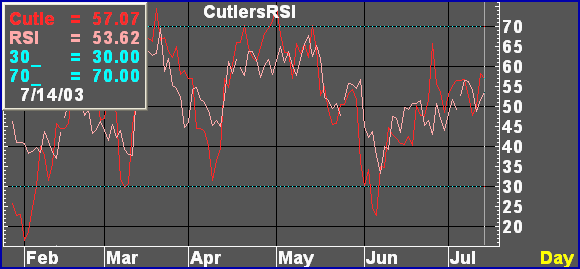# CutlersRSI

 Description Where Wilder's RSI is calculated by determining the difference between the open and the close, Cutler's RSI is calculated by determining the difference between the previous bar's close and the current close (the net change). Formula CutlersRSI(SERIES, Periods=14, Bate=6)=begin   SumUp = 0   SumDown = 0   RS = 1   currentPrice = NONUM   previousPrice = NONUM   difference = 0      for i = -1 to (Periods - 2) begin     currentPrice = GetPrice(\$1, Bate, i, Periods)     previousPrice = GetPrice(\$1, Bate, (i + 1), Periods)     difference = currentPrice - previousPrice     if difference > 0 then SumUp = SumUp + difference     if difference < 0 then SumDown = SumDown + abs(difference)   end   if SumDown > 0 then RS = SumUp / SumDown   retval = GetRS(RS)   retval end Parameters SERIES The SERIES directive makes this formula available as a Formula Study. SERIES refers to the instrument in a chart. SERIES does not become a study parameter.   Periods The number of periods, or length, of the RSI. The default is 14.   Bate The Bate parameter determines what price (open, high, low, last, bid, ask, etc.) is used in calculating. The default is 6, or BATE_CLOSE. Return Value Cutler's RSI ExamplesComments NA